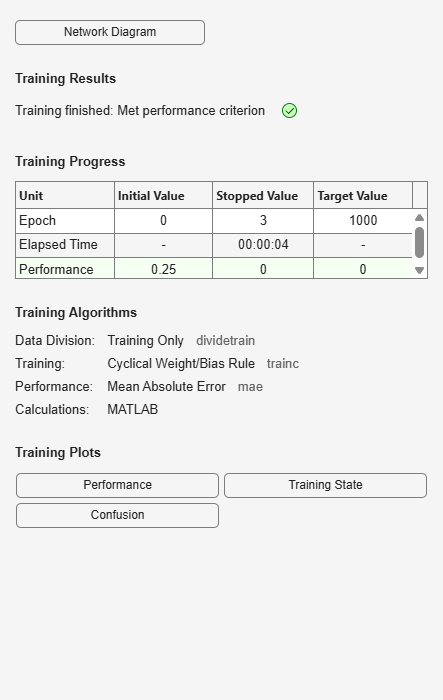# perceptron

Perceptron

## Syntax

```perceptron(hardlimitTF,perceptronLF) ```

## Description

Perceptrons are simple single-layer binary classifiers, which divide the input space with a linear decision boundary.

Perceptrons can learn to solve a narrow range of classification problems. They were one of the first neural networks to reliably solve a given class of problem, and their advantage is a simple learning rule.

`perceptron(hardlimitTF,perceptronLF)` takes these arguments,

 `hardlimitTF` Hard limit transfer function (default = `'hardlim'`) `perceptronLF` Perceptron learning rule (default = `'learnp'`)

and returns a perceptron.

In addition to the default hard limit transfer function, perceptrons can be created with the `hardlims` transfer function. The other option for the perceptron learning rule is `learnpn`.

Note

Deep Learning Toolbox™ supports perceptrons for historical interest. For better results, you should instead use `patternnet`, which can solve nonlinearly separable problems. Sometimes the term “perceptrons” refers to feed-forward pattern recognition networks; but the original perceptron, described here, can solve only simple problems.

## Examples

### Solve Simple Classification Problem Using Perceptron

Use a perceptron to solve a simple classification logical-OR problem.

```x = [0 0 1 1; 0 1 0 1]; t = [0 1 1 1]; net = perceptron; net = train(net,x,t); view(net) y = net(x); ```